# Number of Days in a Month – Formulas in Excel and Google Sheets

This tutorial will teach you how to calculate the number of days in a month using Excel or Google Sheets.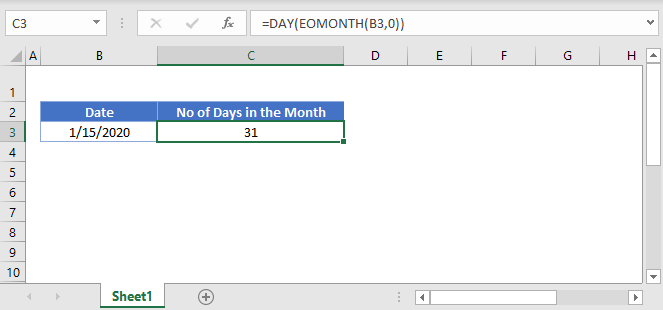## Calculate Number of Days in a Month

### Last Day of Month

The first step to calculating the number of days in a month is to calculate the last day of the month. We can easily do this with the EOMONTH Function:

``=EOMONTH(B3,C3)``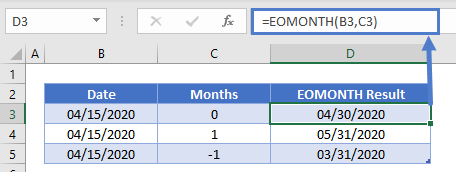Enter the date into the EOMONTH Function and “0” to calculate the last day of the current month (use -1 to calculate the last day of the previous month).

### Calculate Day

Next, use the DAY Function to extract the day number of the last day of the month:

``=DAY(EOMONTH(B3,0))``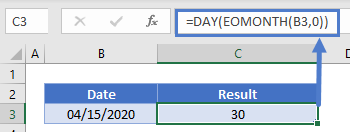This will display the number of days in the month.

## Number of Days in Current Month

Use the previous formula along with the TODAY Function to calculate the last day of the current month:

``=DAY(EOMONTH(TODAY(),0))``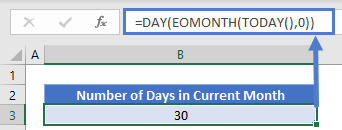## Number of Days in Previous Month

As mentioned above, we set the second argument of the EOMONTH Function to -1 to calculate the last day of the previous month:

``=DAY(EOMONTH(TODAY(),-1))``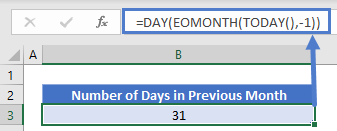## Days in Month – Google Sheets

There formulas work exactly the same in Google Sheets as in Excel: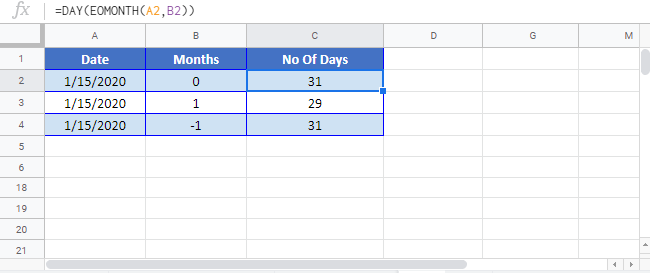### Excel Practice Worksheet

Practice Excel functions and formulas with our 100% free practice worksheets!